>Next: Bibliography

# Measuring Molecular Information

Thomas D. Schneider 1

version = 2.32 of ridebate.tex 1999 November 3
Journal of Theoretical Biology, 201: 87-92, 1999

Although information theory was developed more than 50 years ago [Shannon, 1948,Shannon, 1949], it is widely accepted [Gappmair, 1999], and a complete compendium of Claude Shannon's works was recently published [Sloane & Wyner, 1993]. The application of information theory to understanding binding sites of proteins on DNA or RNA was published more than 10 years ago [Schneider et al., 1986], and since then it has been profitably used to study many genetic systems (see http://www.lecb.ncifcrf.gov// ). Shannon measured information as an average property of signals passing through a communications channel, so a natural extension is to understand the information contributed by individual symbols. The same extension can be applied to the study of binding sites as an individual information theory'' [Schneider, 1997a,Schneider, 1997b] and this has also been successfully used to understand a variety of genetic and medically relevant systems [Hengen et al., 1997,Rogan et al., 1998,Allikmets et al., 1998,,Shultzaberger & Schneider, 1999,Zheng et al., 1999]. Dr. Stormo subsequently published a letter in this journal promoting an alternative to the Shannon approach and pointing out some consequences of that choice [Stormo, 1998]. In this letter I will address other consequences and interpretations of the two approaches. However, before addressing the deep and difficult issues that Dr. Stormo has raised, which we have been discussing for more than 15 years, I would like to make some small factual corrections.

First, the Staden method [Staden, 1984] is discussed in my J. Theor. Biol. paper [Schneider, 1997a]. Staden's method has no cutoff, while the individual information (Ri) method has a natural one and although they are similar, no one derived the Ri formula from Staden's approach. I did not derive the Ri method from Staden; it is a natural extension of information theory inspired by Tribus [Tribus, 1961]. The connection between the information contributed by individual binding sites (as represented by the sequence walker computer graphics [Schneider, 1997b]) and their ensemble average (as represented by the sequence logo computer graphics [Schneider & Stephens, 1990]) is not obvious from the Staden approach, nor is the relationship to energy [Schneider, 1991b].

Second, in his letter [Stormo, 1998] Dr. Stormo implied that I claim an inequality relationship with the enthalpy of binding''. My papers do not claim any relationship with enthalpy; indeed I have not published the word enthalpy'' before now. While it is possible for q in the Second Law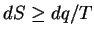to refer to enthalpy (the increase in entropy of the surroundings of a system), the more appropriate measure for molecular machines is the total dissipation, and this corresponds to the free energy. (In this letter I use the terms energy and free energy synonymously.) At this point it would appear that we finally agree, but information is not energy as will be discussed in section II below.

I. What Does Dr. Stormo's Iseq Measure?

1. Iseq is not a state function. Iseq is a relative entropy that is not a distance measure because it is asymmetric and does not follow the triangle inequality [Cover & Thomas, 1991]. So why isn't Iseq a state function? The previous argument used a simple 3 state case [Schneider, 1991b]. A more general argument is to consider a series of N states that form a closed loop. Let N-1 of the steps between these states be made independently so that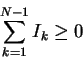(1)

since information'' is (supposedly) additive for each independent event, and each step gives a zero or positive value. Irrespective of whether or not the last step is independent,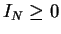because the function is nonnegative. Therefore the sum around a loop is nonnegative: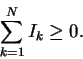(2)

The only condition where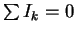is where no step had a change. By making many excursions to different composition regions of the genome, a recognizer would gain an arbitrarily large (and variable) information by Dr. Stormo's measure. In contrast, free energy and entropy are state functions (i.e., functions of the current state of a system and not its history) and so their integration around a closed loop is always zero and prior history can be ignored. Iseqtherefore cannot be used to compute energy as Dr. Stormo claims.

2. Iseq is not an information theory measure. Shannon's uncertainty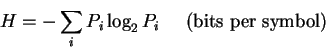(3)

is related to the physical entropy if the probabilities correspond to the microstates of the system, so that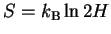[Schneider, 1991b]. (H is often incorrectly called an entropy; see [Tribus & McIrvine, 1971], for an amusing story about why.)

The theory I work with differs from that of Dr. Stormo in that it uses a definition of information that is path independent. A molecular machine -- including not only genetic recognizers but also rhodopsin, myosin, etc. -- dissipates energy into its surroundings as it makes choices [Schneider, 1991a]. The information R(a rate of information, following Shannon's original notation) is a decrease in uncertainty: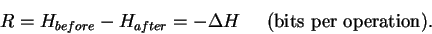(4)

For protein binding on a nucleic acid, the before state is the recognizer unbound or nonspecifically bound and the after state is it being specifically bound [Schneider, 1994]. By using the state function H, the measure R is path independent. As a direct consequence Shannon's information can be compared to the measured energy change of such processes because energy changes are also path independent.

The formula ascribed to by Dr. Stormo is

 Iseq(l) =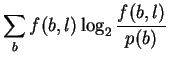=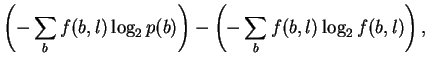(5)

where supposedly p(b) is the probability of base b in the genome, and f(b, l) is the frequency of base b at a position l in a binding site. Writing Iseq in the second form shows that it is a difference, but not of state functions since the first part mixes two states: p(b) represents the unbound state and f(b,l) represents the bound state.

Note that R can be computed from the genomic uncertainty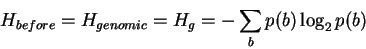(6)

in which case, contrary to Dr. Stormo's claim, it does cancel the background': the information of regions outside a binding site will fluctuate around zero in a sequence logo [Schneider et al., 1986,Schneider & Stephens, 1990]. Therefore equation (6) can account for skewed genome composition. However, this may be fundamentally incorrect as there is no physical contact between the recognizer and the nucleic acid bases in this state.

In other words there are three possible formulas:

 Hbefore = 2 (7) Hbefore = Hg (8)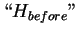=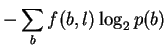(9)

Formula (7) would be the strict molecular machine view in which contact is not made before binding [Schneider, 1991a,Schneider, 1994], so that the uncertainty is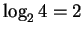bits. This raises the issue of how it is known to be 4 bases. However, the situation is equivalent to determining the channel capacity and therefore follows Shannon in that sense. Modification of bases, for example by methylation or glycosylation, does not increase the information capacity of DNA beyond 2 bits per base since the modifications depend on the sequence itself, for example in the methylation of adenine by Dam methylase at 5' GATC 3'. However, increasing the number of symbols everywhere by adding new bases would increase the information, as has been done experimentally [Piccirilli et al., 1990].

In formula (8) Hg can be used to cancel the background' around a binding site due to genomic composition skew [Schneider et al., 1986], but this is dangerous because we don't know what causes the skew. For example, it could be caused by a nucleosome binding pattern everywhere in the genome and therefore real information is there. This leaves us with the difficult or unresolvable technical problem to separate and identify the information of other binding sites in such genomes. A similar difficult situation is to use purely theoretical means to distinguish ribosome binding site patterns from the downstream codon biases that occur with 3 base repetition. Aside from the toeprint experiment [Hartz et al., 1988] one doesn't know exactly where the 3' edge of the ribosome is [Rudd & Schneider, 1992], and it is not clear that complicated subtraction or extraction schemes would provide fair models close to the initiation codon since translation or protein chains may be different when they are just starting as compared to later on. Experimental approaches to determine the patterns, such as SELEX, are also presently inadequate [Schneider, 1996,Shultzaberger & Schneider, 1999].

Formula (9) is not a true Shannon uncertainty of the form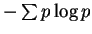, and is not a state function.

Thus formulas (7) or (8) appear reasonable but (9) is not and does not match the physics discussed in Section II.

3. Iseq can violate the channel capacity theorem. Shannon's channel capacity theorem provides an upper bound on the information that can be transmitted [Shannon, 1948,Shannon, 1949]. It has been used to explain the observed precision of molecular systems [Schneider, 1991a,Schneider, 1994]. Because Iseq can give indefinitely large values, it could be used to transmit more information than the channel capacity of a communications system, in violation of the theorem. Dr. Stormo gives an example where more than 2 bits'' per base are obtained from the string GGGG even though it never takes more than 2 bits to choose one object in four.

When discussing the computation for GGGG, Dr. Stormo does not give a justification for having more than 2 bits/base other than having Rsequence (the average information at a set of binding sites) equal Rfrequency (the information needed to locate the binding sites on the genome). There are now a number of clear cases where Rsequencedoes not equal Rfrequency for good biological reasons [Schneider et al., 1986,Schneider & Stormo, 1989,Herman & Schneider, 1992,,Stephens & Schneider, 1992], so forcing one's formula to make them equal means that one could miss important biological phenomena.

4. Interpreting Iseq as a macroscopic measure made by an observer. I understand that it is not Dr. Stormo's intent to model the observational process, but it is worthwhile understanding the implications of this possible interpretation. Formulas like Iseqdirectly compare two probability distributions, and because they always have positive values they can be interpreted as measuring the state change of an observer who doesn't forget. If this is the case, then they are not an appropriate measure for single molecules, which do forget where, or even whether, they were previously bound.

5. Iseq can measure prejudice. Iseq-like functions may be a way of measuring prejudice of an observer. They will give an indefinitely large response when some initial probabilities are small but later turn out to be large (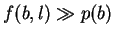in equation 5). That is, the more prejudiced the observer is, the more surprised they can be. This has a curious consequence. If there are 2 possible initial states and an observer believes that one of them is highly likely, then when the states change later the observer can gain more than 1 bit'' of information, even though a 2 state system cannot contain more than 1 bit of information since it takes only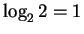yes-no question to completely identify one of the two items. The more prejudiced the person is about the initial state, the more that they learn', and they somehow learn more than it is possible to know! This violation of the channel capacity shows that it is not appropriate to assign the units bits'' to this measure.

6. Iseq as a global free energy measure. Dr. Stormo (private communication) indicates that Iseq is intended to compare two different situations, the protein occurring equally at all possible positions and its equilibrium distribution.'' In other words, Dr. Stormo proposes it as a measure of the macroscopic binding reaction. By this interpretation, Iseq does not measure the state change of a single molecule, so it cannot be used to determine the average energy change a single molecule experiences in the transition between being non-specifically bound to the genome and being bound at the binding sites. The choices made by a single protein cannot be sensitive to the macroscopic chemical equilibrium. For example, the local binding interaction between a single EcoRI molecule and the base A cannot be sensitive to the number of A molecules elsewhere on a DNA. The EcoRI molecule can only react with the bases it is close to.

II. What is the inequality that Dr. Stormo disputes?

The inequality is a version of the Second Law of Thermodynamics, given in a previous J. Theor. Biol. paper [Schneider, 1991b]. The relationship derived from both the Second Law and (surprisingly!) from Shannon's channel capacity equation is: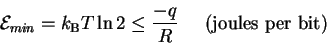(10)

where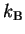is Boltzmann's constant, T is the absolute temperature and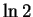is a constant that gives units of bits. Positive q is defined as heat put into the system. The formula shows that to gain one bit of information (set R = 1) at least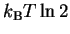joules must be dissipated (-q) to the surroundings. The Second Law forbids a smaller amount but allows a larger amount.

A coin is a useful example for understanding this. A coin can carry one bit of information, since it has 2 states and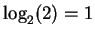bit. Consider a coin flipping in the air or bouncing around in a box. In such a condition it has no particular state and so its uncertainty is 1 bit. To store' information in the coin, it must come to rest on one or the other face. This requires that the energy in the coin be allowed to flow out to the surrounding environment. The point here is that the initial energy of the coin can have different values relative to the final value. The Second Law tells us that there is a minimum energy that must be dissipated per bit (joules), but there can be extra dissipation that is merely wasted because under all conditions no more than 1 bit can be stored in the coin. With even a small inefficiency, the relationship between energy dissipated and information gained will be an inequality, contrary to Dr. Stormo's claim (see [Tribus & McIrvine, 1971]).

A coin is also a good analogy for the situation of a protein binding to DNA. Before specific binding, the protein/DNA complex has high energy, while after binding at specific DNA sites it has lower energy. The excess energy must be dissipated to the surroundings for the molecule to stick, since if the energy were not dissipated the molecule would move on. As with the coin, there can be an excess dissipation so there is no a priori relationship between energy and information aside from the Second Law bound.

If, in attempting to model binding energetics, p(b)and f(b, l)are to represent the time-average of various bases bound by the protein, then the non-equivalence of energy and information means that it is not correct to assume that these are the same as the base frequencies observed in the genome and in binding sites, respectively, since those correspond to information. In this case, these probabilities are not yet experimentally accessible and the measure Dr. Stormo proposes cannot be made.

On the other hand, these probabilities are usually presented as estimatable from observed base frequencies, in which case Dr. Stormo is working entirely on the information side of the energy/information equation (10) to compute his specific free energy of binding''. In this interpretation, Iseq cannot be a measure of energy. Because of the Second Law inequality, the only way to know what the real energy is, is to go and make direct measurements of it.

Acknowledgments

I thank Lakshmanan Iyer for comments and for useful discussions leading to equation (2), and Elaine Bucheimer, John S. Garavelli, Denise Rubens, Peter K. Rogan, John Spouge, Bruce Shapiro, and Ryan Shultzaberger for comments on the manuscript.Next: Bibliography
Tom Schneider
1999-11-03